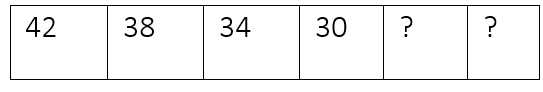Mathematics
Easy

Question

# A subtraction rule was used to make the pattern of numbers in the table.If the pattern continues, then the next two numbers will be

## 28, 24    26, 24    26, 22    24, 20Hint:

## The correct answer is: 26, 22

### The given sequence is 42, 38, 34, 30We have to find the next two terms in this sequence.Let’s find the difference between the first and second term. It will be the constant that is subtracted from all the terms.Constant = first term – second term= 42 – 38= 4So, the difference between each term is 4.Let’s see how it works for every term.38 – 4 = 3434 – 4 = 30Now, let’s find the next terms.The last term from the above sequence is 30So, we will subtract 4 from the term30 – 4 = 26This will be our new first term. We will take this term and subtract 4 to get next term26 – 4 = 22We got the new terms, “26”and “22”. We will see which option matches these values.The complete sequence will be 42, 38, 34, 30, 26, 22And the option which is '26, 22’, is the required option.

For such questions, we should know the basic operations. When the rule used to write the pattern is not mentioned, we have to use all four operations to check the pattern. The operations are addition, subtraction, division, and multiplication.

### Related Questions to study#### With Turito Foundation.#### Get an Expert Advice From Turito.